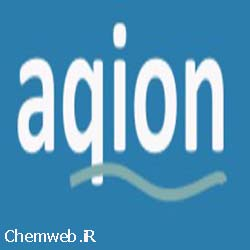October 10, 2021|Aqion software free download is a hydrochemistry software that has many applications in analytical chemistry for the analysis and make solution for chemists.

Key features of Aqion  software:

• validates aqueous solutions: charge balance, EC, …
• calculates pH (acid-base reactions, addition of chemicals)
• calculates the calcite-carbonate system (closed/open CO2 system, LSI)
• calculates alkalinity, buffer capacities (ANC, BNC), and water hardness
• calculates equilibria with mineral phases (dissolution, precipitation, SI)
• calculates and plots titration curves
• calculates redox reactions